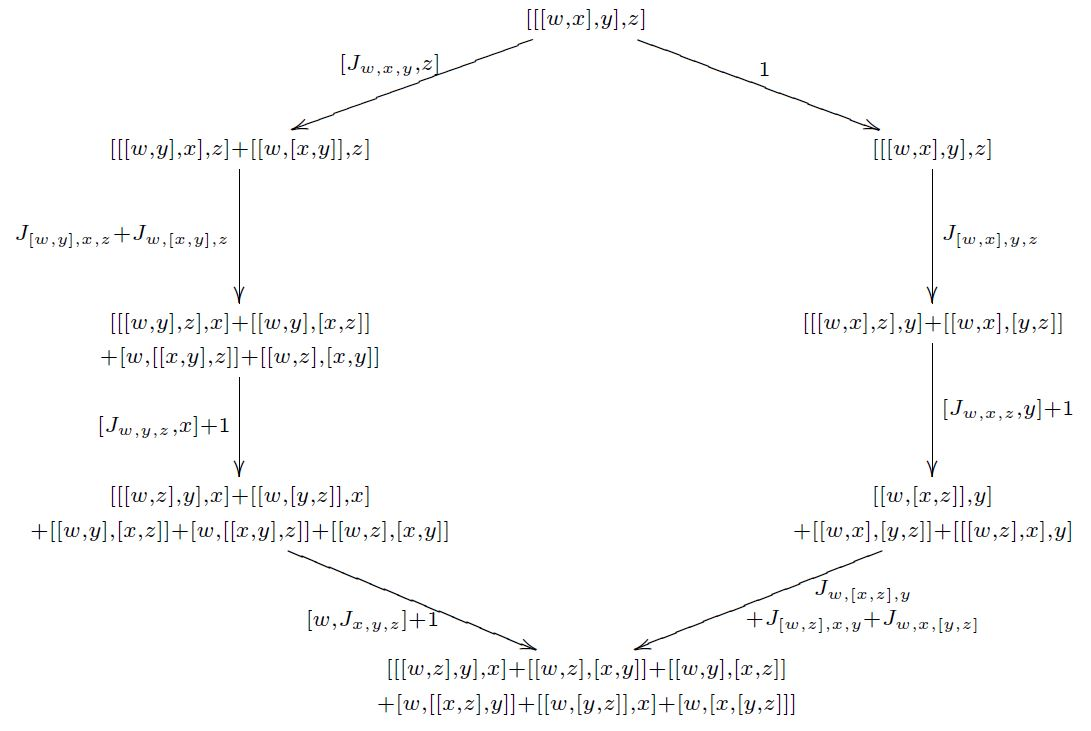Lie $2$-algebras

Idea

A Lie 2-algebra is to a Lie 2-group as a Lie algebra is to a Lie group. Thus, it is a vertical categorification of a Lie algebra.

Definition

Semistrict case

A (“semistrict”) Lie 2-algebra $\mathfrak{g}$ is an L-∞-algebra with generators concentrated in the lowest two degrees.

This means that it is

• a pair of vector spaces $\mathfrak{g}_0, \mathfrak{g}_1$

• equipped with linear functions as follows:

a unary bracket $[-]$ encoding a differential

$\delta : \mathfrak{g}_1 \to \mathfrak{g}_0 \,$

and a binary bracket $[-,-]$, whose component on elements in degree 0 is a Lie bracket

$[-,-] : \mathfrak{g}_0 \vee \mathfrak{g}_0 \to \mathfrak{g}_0$

and whose component on elements in degree 0 and degree 1 is a weak action

$\alpha(-,-) : \mathfrak{g}_0 \otimes \mathfrak{g}_1 \to \mathfrak{g}_1 \,;$

and a trinary bracket

$[-,-,-] : \mathfrak{g}_0 \vee \mathfrak{g}_0 \vee \mathfrak{g}_0 \to \mathfrak{g}_1$

called the Jacobiator;

• such that

• $[-,-]$ and $[-,-,-]$ are skew-symmetric in their arguments, as indicated;

• the differential respects the brackets: for all $x \in \mathfrak{g}_0$ and $h \in \mathfrak{g}_1$ we have

$\delta [x,h] = [x, \delta h]$

hence

$\delta \alpha(x,h) = [x, \delta h] \,;$
• the Jacobi identity of $[-,-]$ holds up to the image under $\delta$ of the Jacobiator $[-,-,-]$: for all $x,y,z \in \mathfrak{g}_0$ we have

$[x,[y,z]] + [y,[z,x]] + [z,[x,y]] = \delta [x,y,z]$
• as does the action property:

$\alpha(x,[y,h]) - \alpha(y,[x,h]) = \alpha([x,y],h) + [x,y,\delta h]$
• the Jacobiator is coherent:

$[[w,x,y], z] + [[w,y,z],x] + [[w,y],x,z] + [[x,z],w,y] = [[w,x,z], y] + [[x,y,z], w] + [[w,x],y,z] + [[w,z], x,y] + [[x,y], w,z] + [[y,z],w,x] \,.$

The Jacobiator identity equivalently expresses the commutativity of the following diagram in the given 2-vector space (analogous to the pentagon identity)(graphics grabbed from Baez-Crans 04, p. 19)

Strict case

If the trinary bracket $[-,-,-]$ in a Lie 2-algebra is trivial, one speaks of a strict Lie 2-algebra. Strict Lie 2-algebras are equivalently differential crossed modules (see there for details).